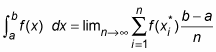##### Differential Equations For Dummies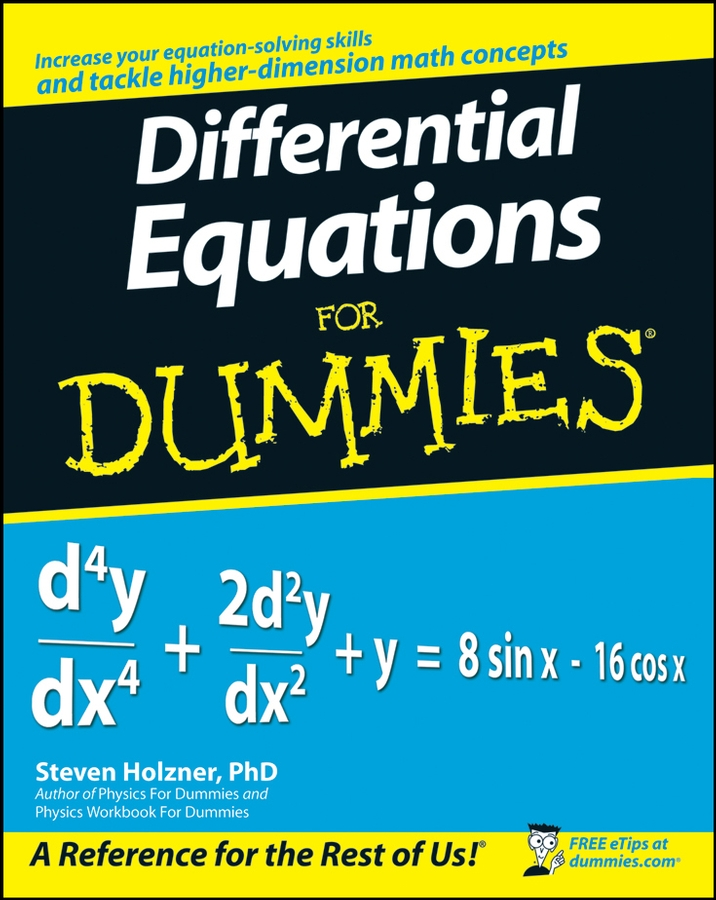The Riemann Sum formula provides a precise definition of the definite integral as the limit of an infinite series. The Riemann Sum formula is as follows: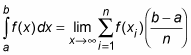Below are the steps for approximating an integral using six rectangles: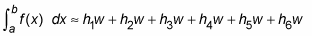1. Increase the number of rectangles (n) to create a better approximation: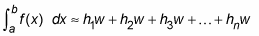2. Simplify this formula by factoring out w from each term: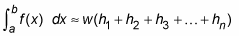3. Use the summation symbol to make this formula even more compact: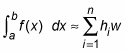The value w is the width of each rectangle: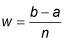Each h value is the height of a different rectangle: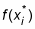So here is the Riemann Sum formula for approximating an integral using n rectangles: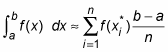4. For a better approximation, use the limit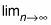5. to allow the number of rectangles to approach infinity: